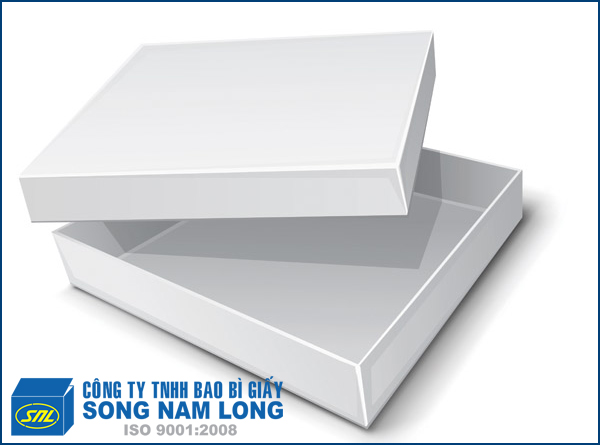In 服务

## 阴阳箱S 底部 = (D+C*2+30)*(R+C*2+30)/1.000.000

S 箱盖 = [((D+10)+(C+10)*2+30)*((R+10)+(C+10)*2+30)]/1.000.000

S阴阳箱=S底部+S箱盖

D  – 箱长

R箱宽

C-箱高

30mm –是纸板两边当生产纸箱之时需要切掉

Recent Posts